# Characteristic Vector Approach to Integrals of Motion Problem

Motivated by our recent work in relativistic fluid dynamics, one insightful approach to the integrals of motion problem involves rewriting the equations of motion in terms of an arbitrary vector field, which we're currently calling a characteristic vector. The benefit here is that we can produce a mathematical condition that tells us what combintations of variables should be grouped together in order to produce conserved quantities. The details follow.

## Newtonian Equations of Motion

Using index summation notation (wherein repeated indices are summed over), it is possible to write the Newtonian equations of motion for a test particle in a fixed, static potential in an arbitrary orthogonal coordinate system. The equation describing motion in the i-dimension is: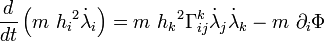$\frac{d}{dt} \left( m \ {h_i}^2 \dot{\lambda}_i \right) = m \ {h_k}^2 \Gamma^k_{ij} \dot{\lambda}_j \dot{\lambda}_k - m \ \partial_i \Phi$

where m is the mass of the test particle, the λs are the chosen coordinates, and the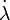$\dot{\lambda}$s are the coordinate velocities (or the total time-derivatives of the coordinates), the hs are the metric scale factors associated with each of the coordinates, Φ is the gravitational potential, and the Γs are the Christoffel symbols.

## Metric Components, Orthogonality Condition, and Scale Factors

For any coordinate system it is possible to express the components of the metric in terms of the partial derivatives defining the transformation from any other coordinate system. Written in terms of the transformation from cylindrical coordinates, each metric component is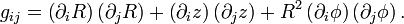$g_{ij} = \left( \partial_i R \right) \left( \partial_j R \right) + \left( \partial_i z \right) \left( \partial_j z \right) + R^2 \left( \partial_i \phi \right) \left( \partial_j \phi \right) .$

The orthogonality condition is that the metric be diagonal. That is, each off-diagonal component of the metric must equal zero.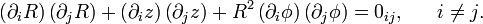$\left( \partial_i R \right) \left( \partial_j R \right) + \left( \partial_i z \right) \left( \partial_j z \right) + R^2 \left( \partial_i \phi \right) \left( \partial_j \phi \right) = 0_{ij}, \ \ \ \ \ i \neq j .$

If the coordinates should be orthogonal, then scale factors can also be defined in terms of the diagonal components of the metric such that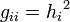$g_{ii} = {h_i}^2$. This results in the following definition of the scale factors if the orthogonality condition is met.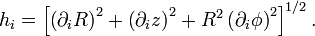$h_i = \left[ \left( \partial_i R \right)^2 + \left( \partial_i z \right)^2 + R^2 \left( \partial_i \phi \right)^2 \right]^{1/2} .$

## Christoffel Symbols

For an orthogonal coordinate system, the Christoffel symbols can be written (depending on which, if any, indices are repeated) as

Christoffel Symbols
(for orthogonal coordinate systems)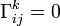$\Gamma^k_{ij} = 0$

for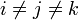$i \ne j \ne k$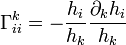$\Gamma^k_{ii} = - \frac{h_i}{h_k} \frac{\partial_k h_i}{h_k}$

for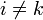$i \ne k$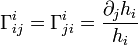$\Gamma^i_{ij} = \Gamma^i_{ji} = \frac{\partial_j h_i}{h_i}$

otherwise
(Mistake in original expression corrected
06/10/2010, as discussed.)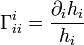$\Gamma^i_{ii} = \frac{\partial_i h_i}{h_i}$

otherwise

## Identification of Conserved Quantity

If, through some miracle, the right-hand side (RHS) of the equation of motion should equal zero, then the combination of variables inside the parentheses must by definition be a conserved quantity. But good luck guessing a coordinate system in which this miracle will occur!

### Introduce Characteristic Vector

The trick is to introduce an arbitrary vector field, which will be used to build a location-dependent weighted linear combination of the equations of motion. Since the equations of motion associated with each of the coordinates collectively form a single vector equation, we will just form an inner product of our characteristic vector with each side of the vector equation of motion. The result is

CV.01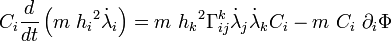$C_i \frac{d}{dt} \left( m \ {h_i}^2 \dot{\lambda}_i \right) = m \ {h_k}^2 \Gamma^k_{ij} \dot{\lambda}_j \dot{\lambda}_k C_i - m \ C_i \ \partial_i \Phi$

Next, we bring Ci inside the total time-derivative on the left-hand side (LHS). This produces an additional term, which we promptly move over to the RHS and include as part of what is commonly referred to as the source (since it's the source of any change in the quantity in parentheses).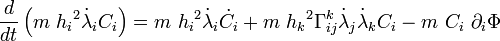$\frac{d}{dt} \left( m \ {h_i}^2 \dot{\lambda}_i C_i \right) = m \ {h_i}^2 \dot{\lambda}_i \dot{C}_i + m \ {h_k}^2 \Gamma^k_{ij} \dot{\lambda}_j \dot{\lambda}_k C_i - m \ C_i \ \partial_i \Phi$

By doing this, we have formed a new conservative quantity; that is, a new quantity in the parentheses which will be conserved if and only if the source is zero. The utility is in the fact that we should be able to force the source associated with this new conservative quantity to go to zero by choosing the right characteristic vector. Once we find the right characteristic vector, we'll be able to use it directly to build the conserved quantity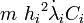$m \ {h_i}^2 \dot{\lambda}_i C_i$.

### Strategic Choice of Characteristic Vector

So we're looking for a vector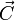$\vec{C}$ such that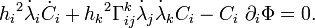${h_i}^2 \dot{\lambda}_i \dot{C}_i + {h_k}^2 \Gamma^k_{ij} \dot{\lambda}_j \dot{\lambda}_k C_i - C_i \ \partial_i \Phi = 0 .$

The good news is that this is a single scalar equation constraining the three independent components of$\vec{C}$, so several families of solutions should exist. Consequently, we can afford to be choosey!

In the problem we're exploring using T3 coordinates, we already know a conserved quantity associated with the azimuthal coordinate — angular momentum. We're looking for an additional (independent) conserved quantity associated with the two radial coordinates. So it makes sense to look for one of the solutions that has C3 = 0. Furthermore, we'd like to avoid dealing with the unknown potential function (which varies only with λ1) as much as possible, so as long as we're being choosey, let's look for a solution that has C1 = 0. We now have three conditions on three components. The condition constraining C2 is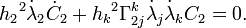${h_2}^2 \dot{\lambda}_2 \dot{C}_2 + {h_k}^2 \Gamma^k_{2j} \dot{\lambda}_j \dot{\lambda}_k C_2 = 0 .$

This can be rewritten more simply as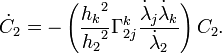$\dot{C}_2 = - \left( \frac{{h_k}^2}{{h_2}^2} \Gamma^k_{2j} \frac{\dot{\lambda}_j \dot{\lambda}_k}{\dot{\lambda}_2} \right) C_2 .$

Perhaps the best approach to solving this condition is separation of variables.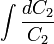$\int \frac{dC_2}{C_2}$ =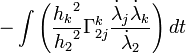$- \int \left( \frac{{h_k}^2}{{h_2}^2} \Gamma^k_{2j} \frac{\dot{\lambda}_j \dot{\lambda}_k}{\dot{\lambda}_2} \right) dt$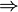$\Rightarrow$ lnC2 =$- \int \left( \frac{{h_k}^2}{{h_2}^2} \Gamma^k_{2j} \frac{\dot{\lambda}_j \dot{\lambda}_k}{\dot{\lambda}_2} \right) dt$$\Rightarrow$ C2 =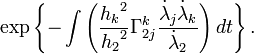$\exp \left\{ - \int \left( \frac{{h_k}^2}{{h_2}^2} \Gamma^k_{2j} \frac{\dot{\lambda}_j \dot{\lambda}_k}{\dot{\lambda}_2} \right) dt \right\} .$

The final necessary step will be to write the quantity in parentheses as the exact derivative of some other quantity, call it Ξ. The long-sought conserved quantity will then be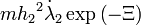$m {h_2}^2 \dot{\lambda}_2 \exp \left( - \Xi \right)$ , where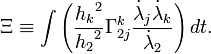$\Xi \equiv \int \left( \frac{{h_k}^2}{{h_2}^2} \Gamma^k_{2j} \frac{\dot{\lambda}_j \dot{\lambda}_k}{\dot{\lambda}_2} \right) dt .$

#### Question from Joel

Is the following logic correct?

Suppose we examine the term in which j = 2 and k = 1. The integral becomes,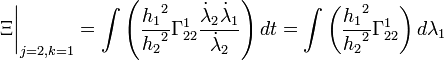$\Xi\biggr|_{j=2,k=1} = \int \left( \frac{{h_1}^2}{{h_2}^2} \Gamma^1_{22} \frac{\dot{\lambda}_2 \dot{\lambda}_1}{\dot{\lambda}_2} \right) dt = \int \left( \frac{{h_1}^2}{{h_2}^2} \Gamma^1_{22} \right) d\lambda_1$

Given that the scale factors and the Christoffel symbols are expressible entirely in terms of λ1 and λ2, one could imagine a situation — for example in the quadratic case of q2 = 2 — in which this integral could be completed analytically. (I presume that wherever λ2 appears inside this integral, it can be treated as a constant because the two coordinates are independent of one another.)

#### Response from Jay

Yes, I believe you've worked this term out correctly, but as you can see in what follows, it cancels out with the other cross term. Writing out each of the terms in Ξ gives,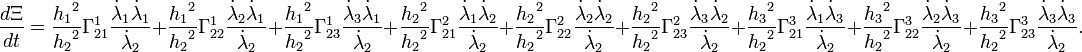$\frac{d\Xi}{dt} = \frac{{h_1}^2}{{h_2}^2} \Gamma^1_{21} \frac{\dot{\lambda}_1 \dot{\lambda}_1}{\dot{\lambda}_2} + \frac{{h_1}^2}{{h_2}^2} \Gamma^1_{22} \frac{\dot{\lambda}_2 \dot{\lambda}_1}{\dot{\lambda}_2} + \frac{{h_1}^2}{{h_2}^2} \Gamma^1_{23} \frac{\dot{\lambda}_3 \dot{\lambda}_1}{\dot{\lambda}_2} + \frac{{h_2}^2}{{h_2}^2} \Gamma^2_{21} \frac{\dot{\lambda}_1 \dot{\lambda}_2}{\dot{\lambda}_2} + \frac{{h_2}^2}{{h_2}^2} \Gamma^2_{22} \frac{\dot{\lambda}_2 \dot{\lambda}_2}{\dot{\lambda}_2} + \frac{{h_2}^2}{{h_2}^2} \Gamma^2_{23} \frac{\dot{\lambda}_3 \dot{\lambda}_2}{\dot{\lambda}_2} + \frac{{h_3}^2}{{h_2}^2} \Gamma^3_{21} \frac{\dot{\lambda}_1 \dot{\lambda}_3}{\dot{\lambda}_2} + \frac{{h_3}^2}{{h_2}^2} \Gamma^3_{22} \frac{\dot{\lambda}_2 \dot{\lambda}_3}{\dot{\lambda}_2} + \frac{{h_3}^2}{{h_2}^2} \Gamma^3_{23} \frac{\dot{\lambda}_3 \dot{\lambda}_3}{\dot{\lambda}_2} .$

Plugging in values for the Christoffel symbols leads to the expression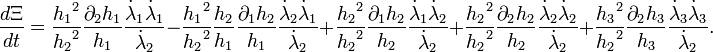$\frac{d\Xi}{dt} = \frac{{h_1}^2}{{h_2}^2} \frac{\partial_2 h_1}{h_1} \frac{\dot{\lambda}_1 \dot{\lambda}_1}{\dot{\lambda}_2} - \frac{{h_1}^2}{{h_2}^2} \frac{h_2}{h_1} \frac{\partial_1 h_2}{h_1} \frac{\dot{\lambda}_2 \dot{\lambda}_1}{\dot{\lambda}_2} + \frac{{h_2}^2}{{h_2}^2} \frac{\partial_1 h_2}{h_2} \frac{\dot{\lambda}_1 \dot{\lambda}_2}{\dot{\lambda}_2} + \frac{{h_2}^2}{{h_2}^2} \frac{\partial_2 h_2}{h_2} \frac{\dot{\lambda}_2 \dot{\lambda}_2}{\dot{\lambda}_2} + \frac{{h_3}^2}{{h_2}^2} \frac{\partial_2 h_3}{h_3} \frac{\dot{\lambda}_3 \dot{\lambda}_3}{\dot{\lambda}_2} .$

And limiting our interest to motion within the meridional plane (setting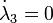$\dot{\lambda}_3 = 0$) and simplifying

CV.02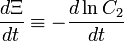$\frac{d\Xi}{dt} \equiv - \frac{d\ln C_2}{dt}$ =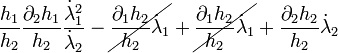$\frac{h_1}{h_2} \frac{\partial_2 h_1}{h_2} \frac{{\dot{\lambda}_1}^2}{\dot{\lambda}_2} - \cancel{\frac{\partial_1 h_2}{h_2} \dot{\lambda}_1} + \cancel{\frac{\partial_1 h_2}{h_2} \dot{\lambda}_1} + \frac{\partial_2 h_2}{h_2} \dot{\lambda}_2$ =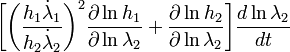$\biggl[ \biggl(\frac{h_1 \dot{\lambda}_1}{h_2 \dot{\lambda}_2}\biggr)^2 \frac{\partial \ln h_1}{\partial\ln\lambda_2} + \frac{\partial \ln h_2}{\partial \ln\lambda_2}\biggr] \frac{d \ln{\lambda}_2}{dt}$ NOTE: Sign error fixed on 15 July 2010. Specifically,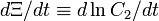$d\Xi/dt \equiv d\ln C_2/dt$ changed to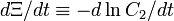$d\Xi/dt \equiv - d\ln C_2/dt$.

# Application to T3 Coordinates

On a separate page, we have applied this "characteristic vector" approach specifically to T3 coordinates.

 © 2014 - 2020 by Joel E. Tohline |   H_Book Home   |   YouTube   | Appendices: | Equations | Variables | References | Ramblings | Images | myphys.lsu | ADS |